﻿• 价格透明
• 信息保密
• 进度掌控
• 售后无忧# 使用Resample函数转换时间序列

## 一、什么是resample函数？

11月1号周一1.111.111.111.12
11月2号周二1.121.121.111.12
11月3号周三1.131.131.111.12
11月4号周四1.151.141.111.12
11月5号周五1.141.151.111.12

## 二、实战Resample函数

### 1.日K 转换为 周K

#### 1.1函数文档学习

https://pandas.pydata.org/pandas-docs/stable/reference/api/pandas.DataFrame.resample.html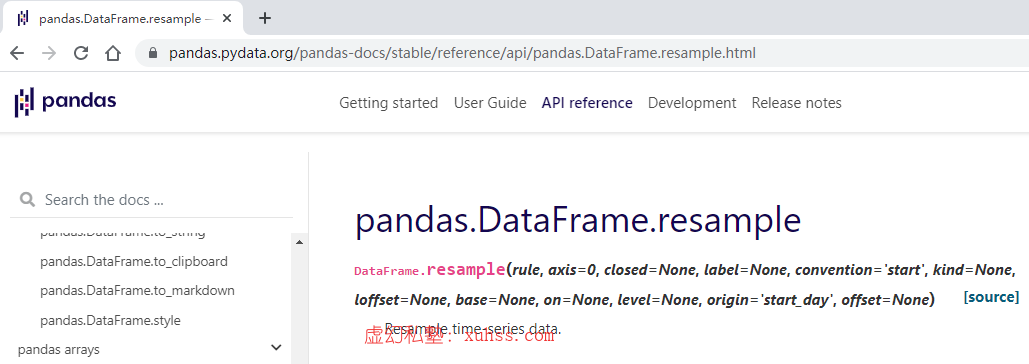• rule表示的是你放一个什么样的周期性指标在里面，用m代表Month，Y代表Year,w代表Week，
• closed代表你取哪一个分界线，举例来说，比如说我把日k转换为周k，到底我是取周一为分界线还是周五为分界线呢？这就是通过closed来确定的。

``````>>>index = pd.date_range('1/1/2000', periods=9, freq='T')
>>>series = pd.Series(range(9), index=index)
>>>series
2000-01-01 00:00:00    0
2000-01-01 00:01:00    1
2000-01-01 00:02:00    2
2000-01-01 00:03:00    3
2000-01-01 00:04:00    4
2000-01-01 00:05:00    5
2000-01-01 00:06:00    6
2000-01-01 00:07:00    7
2000-01-01 00:08:00    8
Freq: T, dtype: int64
``````

``````>>>series.resample('3T').sum()
2000-01-01 00:00:00     3
2000-01-01 00:03:00    12
2000-01-01 00:06:00    21
Freq: 3T, dtype: int64
``````

``````>>>series.resample('3T', label='right').sum()
2000-01-01 00:03:00     3
2000-01-01 00:06:00    12
2000-01-01 00:09:00    21
Freq: 3T, dtype: int64
``````

#### 1.2实战

``````#获取日k
df = get_price("000001.XSHG", end_date='2021-05-30 14:00:00',count=20, frequency='1d', fields=['open','close','high','low','volume','money'])
print(df)
``````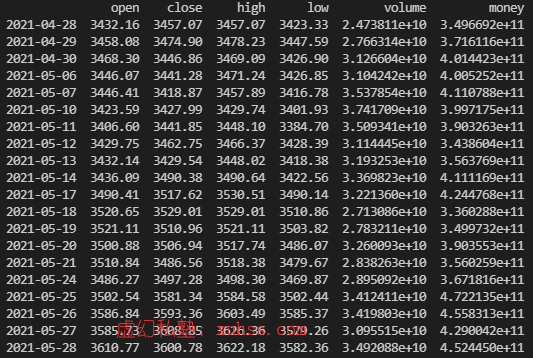``````#获取日k
df = get_price("000001.XSHG", end_date='2021-05-30 14:00:00',count=20, frequency='1d', fields=['open','close','high','low','volume','money'])
df['weekday']=df.index.weekday
print(df)
``````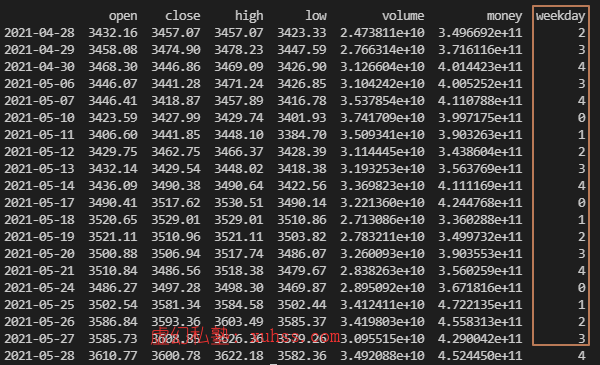``````#获取周k
import pandas as pd
df_week = pd.DataFrame()
df_week = df['open'].resample('W').first()
print(df_week)
``````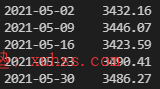``````#获取周k
import pandas as pd
df_week = pd.DataFrame()
df_week['open'] = df['open'].resample('W').first()
df_week['close'] = df['close'].resample('W').last()
df_week['high'] = df['high'].resample('W').max()
df_week['low'] = df['low'].resample('W').min()
print(df_week)
``````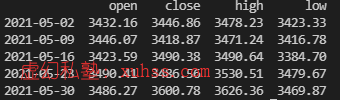### 2.汇总统计功能（统计月成交量、成交额）

``````#获取周k
import pandas as pd
df_week = pd.DataFrame()
df_week['open'] = df['open'].resample('W').first()
df_week['close'] = df['close'].resample('W').last()
df_week['high'] = df['high'].resample('W').max()
df_week['low'] = df['low'].resample('W').min()
df_week['volume(sum)'] = df['volume'].resample('W').sum()
df_week['money(sum)'] = df['money'].resample('W').sum()
print(df_week)
``````

### 3.日K 转换为 月K

1. 添加`start_date`获取到一整年的数据
2. `resample`的参数改为M即可，M代表Month
``````#获取日k
df = get_price("000001.XSHG", end_date='2021-05-30 14:00:00', start_date='2020-05-30', frequency='1d', fields=['open','close','high','low','volume','money'])
df['weekday']=df.index.weekday
print(df)

#获取周k
import pandas as pd
df_week = pd.DataFrame()
df_week['open'] = df['open'].resample('M').first()
df_week['close'] = df['close'].resample('M').last()
df_week['high'] = df['high'].resample('M').max()
df_week['low'] = df['low'].resample('M').min()
print(df_week)
``````### 低价透明### 金牌服务### 信息保密